### Applying the "rule of 70" to growth rate problems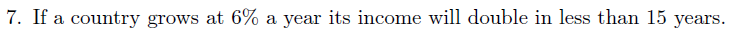True or Flase?Equations on page 45 & 49 of Jones Macro Econ Crisis Update Edition. ~ Moral of the story, be ready to show how you would solve this problem with a calculator, and be ready for the arithmetic of dividing things by 70. Method 1) If I had a calculator I'd use the following formula: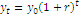however, you'll likely not be able to use a calc. How many years to double at 6% growth?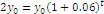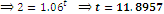And 11.8957 is less than 15 years, so true. But, alas, fie cruel world, Aspen will not allow you to use a calculator on the exam. Method 2) Use the ‘rule of 70’ (it’s actually the rule of 72, if you are good at long division, use the rule of 72). No calculator should be needed to implement the Rule of 70: How many years to double at 6% growth?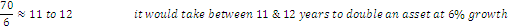Thus, the answer is clearly true! Method 3) Also, let’s say the question was worded slightly different and you needed to know the percentage rate that would double an asset in 15 years, you could do the following;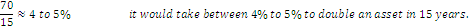Comments